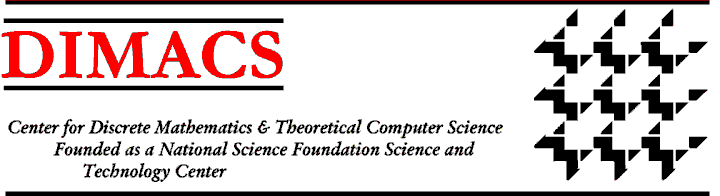### DIMACS Workshop on Properties of Large Graphs: From Combinatorics to Statistical Physics and Back

#### October 16 - 20, 2006 DIMACS Center, CoRE Building, Rutgers University

Organizers:
László Lovász, Microsoft Research and Eötvös Loránd University, lovasz@cs.elte.hu
Benny Sudakov, Princeton University, bsudakov@math.princeton.edu
Presented under the auspices of the Special Focus on Discrete Random Systems.

Many topics of research in mathematics, computer science and physics involve properties of very large graphs, or of graph sequences that grow asymptotically. For example, such topics arise in the study of Internet models, quasi-random graphs and other questions in graph theory, in property testing of large graphs, and in statistical mechanics. One of the areas of mathematics where these questions have been studied extensively is extremal graph theory. Given the central question in extremal graph theory concerning the existence of various small subgraphs, it might at first seem that this area has little to do with the other themes of this workshop, which are all probabilistic in nature. It turns out, however, that this is not the case. Indeed, one of the central results in this area is Szemerédi's Regularity Lemma, which has connections to, e.g., property testing and so-called quasi-random graphs. Quasi-random (also called pseudo-random) graphs were introduced by Thomason and Chung, Graham and Wilson. These graphs are deterministic, but are similar in many ways to random graphs: they have the same number of small subgraphs as a truly random graph, a partition into large subgraphs has similar cuts (number of edges between the different parts of the partitions), etc. In fact, these different characterizations have been proven to be equivalent.

Recently, a program has begun to generalize the results from quasi-random graphs to a large class of graph sequences . The work was originally motivated by an attempt to understand in which sense scale-free graphs like the Barabasi-Albert graph tend to a limit as their size goes to infinity, but turns out to have much wider scope. People working on this new program include researchers coming from many areas, including topology (Michael Freedman), group theory (Balazs Szegedy), combinatorics and computer science (Jeff Kahn, Laci Lovász, Lex Schrijver, Vera Sos, Katalin Vesztergombi) and probability theory and mathematical statistical physics (Christian Borgs, Jennifer Chayes).

One of the central notions in this program is the notion of a convergent graph sequence G n. For dense graphs, one of the many equivalent definitions requires that for any finite graph F on k vertices, the probability that an induced subgraph on k random vertices in G n is isomorphic to F tends to a limit as n → ∞.

Other equivalent notions involve uniform Szemerédi partitions, the number of graph homomorphisms from Gn into weighted graphs H, properties of small random subgraphs, convergence of the adjacency matrix to a measurable function on [0,1] x [0,1], and so on. Each of these definitions carries connections to other fields: graph homomorphisms are related to partition functions in statistical physics, small random subgraphs are related to property testing, and other concepts in this new approach lead to a better understanding of some of the results in extremal graph theory, to mention just a few.

The workshop will bring together researchers from the fields of graph homomorphisms, extremal graph theory, quasi-random graphs, property testing, Internet modeling, probability theory and statistical physics (as practiced by mathematical physicists as well as theoretical physicists).Next: Call for ParticipationWorkshop IndexDIMACS Homepage
Contacting the Center